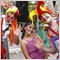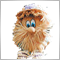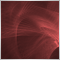29

hi new mql5 developer here

I have 2 errors can you help me?

'{' - function definition unexpected 4MA.mq5 462 1

'{' - function definition unexpected 4MA.mq5 471 1

//+------------------------------------------------------------------+

//|                                              4matendlingline.mq5 |

//|                     Copyright 2022, Client;st james exchange hub |

//|                                                    stjamesxh.com |

//+------------------------------------------------------------------+

#property version   "1.00"
#property description   "This expert advisor is that use 4 MAs"
#property description   "EMA 90"
#property description   "DEMA 38"
#property description   "HMA 9"
#property description   "SMA 9"

//+------------------------------------------------------------------+
//| Include                                                          |
//+------------------------------------------------------------------+

//--- variable

CPositionInfo  m_position;                   // trade position object
CSymbolInfo    m_symbol;                     // symbol info object
CAccountInfo   m_account;                    // account info wrapper

//+------------------------------------------------------------------+
//| Inputs                                                           |
//+------------------------------------------------------------------+
input int                 emaPeriod         =90;
input ENUM_APPLIED_PRICE  emaAppliedPrice   =PRICE_CLOSE;
input int                 demaPeriod        =38;
input ENUM_APPLIED_PRICE  demaAppliedPrice  =PRICE_CLOSE;
input int                 hmaPeriod         =9;
input ENUM_APPLIED_PRICE  hmaAppliedPrice   =PRICE_CLOSE;
input int                 smaPeriod         =9;
input ENUM_APPLIED_PRICE  smaAppliedPrice   =PRICE_WEIGHTED;
input int                 Slippage          =10;

//+------------------------------------------------------------------+
//| Expert initialization function
//+------------------------------------------------------------------+
int OnInit(void)
{
// Sets symbol name
if(!m_symbol.Name(_Symbol)){
Print(__FUNCTION__," Unable to set symbol name.");
return INIT_FAILED;
}

m_trade.SetDeviationInPoints   ( Slippage        );    // Set Daviation(tolerance to price changes during order execution)

//---
return(INIT_SUCCEEDED);
}

//+------------------------------------------------------------------+
//| Expert tick function
//+------------------------------------------------------------------+
void OnTick(void)
{
bool   IsNewCandle =false;
double DEMA_1      =0.0,
DEMA_2      =0.0,
EMA         =0.0,
HMA_1       =0.0,
HMA_2       =0.0,
SMA_1       =0.0,
SMA_2       =0.0;

// Get MA values
EMA    =GetValueEMA(1);                              // Red    90
DEMA_1 =GetValueDEMA(1); DEMA_2  =GetValueDEMA(2);   // Orange 38
HMA_1  =GetValueHMA(1);  HMA_2   =GetValueHMA(2);    // Black  9
SMA_1  =GetValueSMA(1);  SMA_2   =GetValueSMA(2);    // Pink   9

// Allow only if there is a new candle
IsNewCandle=OnNewCandle(PERIOD_CURRENT);
if(!IsNewCandle){
return;
}

// Main logic
// HMA crosses SMA upwards and above red line    (1)
if((HMA_2 < SMA_2 && HMA_1 > SMA_1) && (HMA_1 > EMA && SMA_1 > EMA))
{
Print("HMA crosses SMA upwards and above red line");
}
// HMA crosses SMA downwords and above red line  (2)
else if((HMA_2 > SMA_2 && HMA_1 < SMA_1) && (HMA_1 > EMA && SMA_1 > EMA))
{
PositionOpen(POSITION_TYPE_SELL,0.01,0,0,0);
Print("HMA crosses SMA downwords and above red line");
}
// HMA crosses SMA upwards and below red line    (3)
else if((HMA_2 < SMA_2 && HMA_1 > SMA_1) && (HMA_1 < EMA && SMA_1 < EMA))
{
Print("HMA crosses SMA upwards and below red line");
}
// HMA crosses SMA downwords and below red line  (4)
else if((HMA_2 > SMA_2 && HMA_1 < SMA_1) && (HMA_1 < EMA && SMA_1 < EMA))
{
PositionOpen(POSITION_TYPE_SELL,0.02,0,0,0);
Print("HMA crosses SMA downwords and below red line");
}
// HMA crosses DEMA upwards and above red line   (5)
else if((HMA_2 < DEMA_2 && HMA_1 > DEMA_1) && (HMA_1 > EMA && DEMA_1 > EMA))
{
Print("HMA crosses DEMA upwards and above red line");
}
// HMA crosses DEMA downwords and above red line (6)
else if((HMA_2 > DEMA_2 && HMA_1 < DEMA_1) && (HMA_1 > EMA && DEMA_1 > EMA))
{
PositionOpen(POSITION_TYPE_SELL,0.01,0,0,0);
Print("HMA crosses DEMA downwords and above red line");
}
// HMA crosses DEMA upwards and below red line   (7)
else if((HMA_2 < DEMA_2 && HMA_1 > DEMA_1) && (HMA_1 < EMA && DEMA_1 < EMA))
{
Print("HMA crosses DEMA upwards and below red line");
}
// HMA crosses DEMA downwords and below red line (8)
else if((HMA_2 > DEMA_2 && HMA_1 < DEMA_1) && (HMA_1 < EMA && DEMA_1 < EMA))
{
PositionOpen(POSITION_TYPE_SELL,0.02,0,0,0);
Print("HMA crosses DEMA downwords and below red line");
}
}

//+------------------------------------------------------------------+
//| Expert deinitialization function
//+------------------------------------------------------------------+
void OnDeinit(const int reason)
{

}
//+------------------------------------------------------------------+
//| Return DEMA value
//+------------------------------------------------------------------+
double GetValueDEMA(int shift)
{
int      DEMA_handle =INVALID_HANDLE;
double   DEMA_buffer[];

DEMA_handle =iDEMA(Symbol(), PERIOD_M15, demaPeriod, 0, demaAppliedPrice);
if(DEMA_handle==INVALID_HANDLE)
{
Print("Failed to create handle of the DEMA indicator. err=",GetLastError());
return 0.0;
}

ArraySetAsSeries(DEMA_buffer, true);

if(CopyBuffer(DEMA_handle, 0, 0, 20, DEMA_buffer)<=0)
return 0.0;

return NormalizeDouble(DEMA_buffer[shift],Digits());
}
//
//+------------------------------------------------------------------+
//| Return EMA value
//+------------------------------------------------------------------+
double GetValueEMA(int shift)
{
int      EMA_handle =INVALID_HANDLE;
double   EMA_buffer[];

EMA_handle =iMA(Symbol(), PERIOD_M5, emaPeriod, 0, MODE_EMA, emaAppliedPrice);
if(EMA_handle==INVALID_HANDLE)
{
Print("Failed to create handle of the EMA indicator. err=",GetLastError());
return 0.0;
}

ArraySetAsSeries(EMA_buffer, true);

if(CopyBuffer(EMA_handle, 0, 0, 20, EMA_buffer)<=0)
return 0.0;

return NormalizeDouble(EMA_buffer[shift],Digits());
}
//
//+------------------------------------------------------------------+
//| Return HMA value
//+------------------------------------------------------------------+
{
int      HMA_handle =INVALID_HANDLE;
double   HMA_buffer[];
double   devisor=2.0;

HMA_handle =iCustom(Symbol(), PERIOD_M5, "Hull average.ex5", hmaPeriod, devisor, hmaAppliedPrice);
if(HMA_handle==INVALID_HANDLE)
{
Print("Failed to create handle of the HMA indicator. err=",GetLastError());
return 0.0;
}

ArraySetAsSeries(HMA_buffer, true);

if(CopyBuffer(HMA_handle, 0, 0, 20, HMA_buffer)<=0)
return 0.0;

return NormalizeDouble(HMA_buffer[shift],Digits());
}
//
//+------------------------------------------------------------------+
//| Return SMA value
//+------------------------------------------------------------------+
double GetValueSMA(int shift)
{
int      SMA_handle =INVALID_HANDLE;
double   SMA_buffer[];

//---
SMA_handle =iMA(Symbol(), PERIOD_M5, smaPeriod, 0, MODE_SMA, smaAppliedPrice);
if(SMA_handle==INVALID_HANDLE)
{
Print("Failed to create handle of the SMA indicator. err=",GetLastError());
return 0.0;
}

ArraySetAsSeries(SMA_buffer, true);

if(CopyBuffer(SMA_handle, 0, 0, 20, SMA_buffer)<=0)
return 0.0;

//---
return NormalizeDouble(SMA_buffer[shift],Digits());
}

//+------------------------------------------------------------------+
//|                                                                  |
//+------------------------------------------------------------------+
bool hma_crosses_sma_Upward(int shift)
{
bool hma_crosses_sma_Upward = false;

if(GetValueHMA(shift + 1) < GetValueSMA(shift + 1) && GetValueHMA(shift) > GetValueSMA(shift)) // hma crosses sma upwards

{

hma_crosses_sma_Upward = true;

}

return (hma_crosses_sma_Upward);
}

//+------------------------------------------------------------------+
//|                                                                  |
//+------------------------------------------------------------------+
bool hma_crosses_sma_Downward(int shift)
{
bool hma_crosses_sma_Downward = false;

if(GetValueHMA(shift + 1) > GetValueSMA(shift + 1) && GetValueHMA(shift) < GetValueSMA(shift)) // hma crosses sma upwards

{

hma_crosses_sma_Downward = true;

}

return (hma_crosses_sma_Downward);
}

//+------------------------------------------------------------------+
//|                                                                  |
//+------------------------------------------------------------------+
bool hma_crosses_dema_Upward(int shift)
{
bool hma_crosses_dema_Upward = false;

if(GetValueHMA(shift + 1) < GetValueDEMA(shift + 1) && GetValueHMA(shift) > GetValueDEMA(shift)) // hma crosses dema upwards

{

hma_crosses_dema_Upward = true;

}

return (hma_crosses_dema_Upward);
}

//+------------------------------------------------------------------+
//|                                                                  |
//+------------------------------------------------------------------+
bool hma_crosses_dema_Downward(int shift)
{
bool hma_crosses_dema_Downward = false;

if(GetValueHMA(shift + 1) > GetValueDEMA(shift + 1) && GetValueHMA(shift) < GetValueDEMA(shift)) // hma crosses dema upwards

{

hma_crosses_dema_Downward = true;

}

return (hma_crosses_dema_Downward);
}
//+------------------------------------------------------------------+
//| New candle check function
//+------------------------------------------------------------------+
bool OnNewCandle(const ENUM_TIMEFRAMES PrmTF)
{
static datetime previous_time=0;
datetime current_time=iTime(Symbol(), PrmTF, 0);
if(current_time!=previous_time){
previous_time=current_time;
return true;
}
//---
return false;
}
//+------------------------------------------------------------------+
//| Open position
//+------------------------------------------------------------------+
bool PositionOpen(const ENUM_POSITION_TYPE  PrmType,
const double              PrmLot,
const uint                PrmSL,
const uint                PrmTP,
const ushort              PrmMagic
)
{
int    send        =-1,
stop_level  =-1,
err         =-1;
long   magic       =-1;
Bid         =0.0,
price       =0.0,
pos_sl      =0.0,
pos_tp      =0.0;
string comment     ="",
symbol      ="";

// Refersh data
m_symbol.RefreshRates();
m_symbol.Refresh();

//---
ResetLastError();

//---
Bid        =m_symbol.Bid();
stop_level =m_symbol.StopsLevel();
magic      =(long)PrmMagic;
comment    ="4MA";

// Protection against the return value of "zero"
if(Bid == 0.0) Bid = SymbolInfoDouble(Symbol(), SYMBOL_BID);

/***** Note ******/
// Buying is done at the Ask price — the TakeProfit and StopLoss levels should be compared to the Bid price.
// Selling is done at the Bid price — the TakeProfit and StopLoss levels should be compared to the Ask price.

//---
symbol =m_symbol.Name();
if(symbol==""){
symbol=Symbol();
}

//---
{
/* Debugging */

// Check stop level for SL in buy case
if(PrmSL>0){
pos_sl = NormalizeDouble(pos_sl,Digits());
}
// Check stop level for TP in buy case
if(PrmTP>0){
pos_tp = NormalizeDouble(pos_tp,Digits());
}
if(!send)
{
err=GetLastError();
Print(__LINE__,": Unable to open buy position. error '",err);
return false;
}
}
if(PrmType==POSITION_TYPE_SELL) // If sell selected
{
/* Debugging */
//Print("PrmSL=",PrmSL,"  stop_level=",stop_level,"  Bid=",Bid);

// Check stop level for SL in sell case
if(PrmSL>0){
pos_sl = (PrmSL>(uint)stop_level) ? Bid+PrmSL*Point() : Bid-stop_level*Point();
pos_sl = NormalizeDouble(pos_sl,Digits());
}
// Check stop level for TP in sell case
if(PrmTP>0){
pos_tp = (PrmTP>(uint)stop_level) ? Bid-PrmTP*Point() : Bid+stop_level*Point();
pos_tp = NormalizeDouble(pos_tp,Digits());
}
// Open sell position
if(!send)
{
err=GetLastError();
Print(__LINE__,": Unable to open sell position. error '",err);
return false;
}
}
//---
return true;
}
//+------------------------------------------------------------------+

#property indicator_chart_window
#property indicator_buffers 2
#property indicator_plots   1
#property indicator_label1  "Hull"
#property indicator_type1   DRAW_COLOR_LINE
#property indicator_color1  clrGray,clrMediumSeaGreen,clrOrangeRed
#property indicator_width1  2

//
//
//
//
//

input int                inpPeriod  = 20;          // Period
input double             inpDivisor = 2.0;         // Divisor ("speed")
input ENUM_APPLIED_PRICE inpPrice   = PRICE_CLOSE; // Price

double val[],valc[];

//------------------------------------------------------------------
//
//------------------------------------------------------------------
//
//
//

{

SetIndexBuffer(0,val,INDICATOR_DATA);
SetIndexBuffer(1,valc,INDICATOR_COLOR_INDEX);
iHull.init(inpPeriod,inpDivisor);
IndicatorSetString(INDICATOR_SHORTNAME,"Hull ("+(string)inpPeriod+")");
return (INIT_SUCCEEDED);
}

{
}

//------------------------------------------------------------------
//
//------------------------------------------------------------------
//
//
//
//
//

int OnCalculate(const int rates_total,const int prev_calculated,const datetime &time[],
const double &open[],
const double &high[],
const double &low[],
const double &close[],
const long &tick_volume[],
const long &volume[],
{
int i= prev_calculated-1; if (i<0) i=0; for (; i<rates_total && !_StopFlag; i++)
{
val[i]  = iHull.calculate(getPrice(inpPrice,open,high,low,close,i),i,rates_total);
valc[i] = (i>0) ? (val[i]>val[i-1]) ? 1 : (val[i]<val[i-1]) ? 2 : valc[i-1] : 0;
}
return(i);
}

//------------------------------------------------------------------
// Custom function(s)
//------------------------------------------------------------------
//
//---
//

class CHull
{
private :
int    m_fullPeriod;
int    m_halfPeriod;
int    m_sqrtPeriod;
int    m_arraySize;
double m_weight1;
double m_weight2;
double m_weight3;
struct sHullArrayStruct
{
double value;
double value3;
double wsum1;
double wsum2;
double wsum3;
double lsum1;
double lsum2;
double lsum3;
};
sHullArrayStruct m_array[];

public :
CHull() : m_fullPeriod(1), m_halfPeriod(1), m_sqrtPeriod(1), m_arraySize(-1) {                     }
~CHull()                                                                      { ArrayFree(m_array); }

///
///
///

bool init(int period, double divisor)
{
m_fullPeriod = (int)(period>1 ? period : 1);
m_halfPeriod = (int)(m_fullPeriod>1 ? m_fullPeriod/(divisor>1 ? divisor : 1) : 1);
m_sqrtPeriod = (int) MathSqrt(m_fullPeriod);
m_arraySize  = -1; m_weight1 = m_weight2 = m_weight3 = 1;
return(true);
}

//
//
//

double calculate( double value, int i, int bars)
{
if (m_arraySize<bars) { m_arraySize = ArrayResize(m_array,bars+500); if (m_arraySize<bars) return(0); }

//
//
//

m_array[i].value=value;
if (i>m_fullPeriod)
{
m_array[i].wsum1 = m_array[i-1].wsum1+value*m_halfPeriod-m_array[i-1].lsum1;
m_array[i].lsum1 = m_array[i-1].lsum1+value-m_array[i-m_halfPeriod].value;
m_array[i].wsum2 = m_array[i-1].wsum2+value*m_fullPeriod-m_array[i-1].lsum2;
m_array[i].lsum2 = m_array[i-1].lsum2+value-m_array[i-m_fullPeriod].value;
}
else
{
m_array[i].wsum1 = m_array[i].wsum2 =
m_array[i].lsum1 = m_array[i].lsum2 = m_weight1 = m_weight2 = 0;
for(int k=0, w1=m_halfPeriod, w2=m_fullPeriod; w2>0 && i>=k; k++, w1--, w2--)
{
if (w1>0)
{
m_array[i].wsum1 += m_array[i-k].value*w1;
m_array[i].lsum1 += m_array[i-k].value;
m_weight1        += w1;
}
m_array[i].wsum2 += m_array[i-k].value*w2;
m_array[i].lsum2 += m_array[i-k].value;
m_weight2        += w2;
}
}
m_array[i].value3=2.0*m_array[i].wsum1/m_weight1-m_array[i].wsum2/m_weight2;

//
//---
//

if (i>m_sqrtPeriod)
{
m_array[i].wsum3 = m_array[i-1].wsum3+m_array[i].value3*m_sqrtPeriod-m_array[i-1].lsum3;
m_array[i].lsum3 = m_array[i-1].lsum3+m_array[i].value3-m_array[i-m_sqrtPeriod].value3;
}
else
{
m_array[i].wsum3 =
m_array[i].lsum3 = m_weight3 = 0;
for(int k=0, w3=m_sqrtPeriod; w3>0 && i>=k; k++, w3--)
{
m_array[i].wsum3 += m_array[i-k].value3*w3;
m_array[i].lsum3 += m_array[i-k].value3;
m_weight3        += w3;
}
}
return(m_array[i].wsum3/m_weight3);
}
};
CHull iHull;

//
//---
//

template <typename T>
double getPrice(ENUM_APPLIED_PRICE tprice, T& open[], T& high[], T& low[], T& close[], int i)
{
switch(tprice)
{
case PRICE_CLOSE:     return(close[i]);
case PRICE_OPEN:      return(open[i]);
case PRICE_HIGH:      return(high[i]);
case PRICE_LOW:       return(low[i]);
case PRICE_MEDIAN:    return((high[i]+low[i])/2.0);
case PRICE_TYPICAL:   return((high[i]+low[i]+close[i])/3.0);
case PRICE_WEIGHTED:  return((high[i]+low[i]+close[i]+close[i])/4.0);
}
return(0);
}
//------------------------------------------------------------------Moderator
291613

EA opens Many Trades, How to Open 1 buy & 1 sell?

You have a huge mistake - you create an indicator handle at every tick.

REMEMBER: The MQL5 style implies that the indicator handle MUST be created ONLY ONCE and this must be done in OnInit !!!6680

burcu kıratlı:

hi new mql5 developer here

I have 2 errors can you help me?

'{' - function definition unexpected 4MA.mq5 462 1

'{' - function definition unexpected 4MA.mq5 471 1

exactly as the error says you have not provided any definition to the functions

the first looks like the code should belong in OnInit based upon the return code

the second is empty so delete it

you are probably in the mess because you have not laid your code out very well with bits and pieces everywhere

{

SetIndexBuffer(0,val,INDICATOR_DATA);
SetIndexBuffer(1,valc,INDICATOR_COLOR_INDEX);
iHull.init(inpPeriod,inpDivisor);
IndicatorSetString(INDICATOR_SHORTNAME,"Hull ("+(string)inpPeriod+")");
return (INIT_SUCCEEDED);
}

{
}